## 2012年9月17日星期一

### Fate/Legco: 機器學習與選舉工程

\begin{align*} &\phantom{=}李永達與\ 9\ 位當選者的總得票率\\ &= \frac1{10} + p_1 + \ldots + p_9\\ &> \frac1{10} + \underbrace{\frac1{10} + \ldots + \frac1{10}}_{9 個} \quad（因為每個\ p_i\ 都大過 \frac1{10}）\\ &= 1. \end{align*}

$d_1(n)=\frac1{n+1}$ 的票，不管其他對手取得幾多票，此君亦鐵定當選，否則就會出現「部份候選人的得票率總和竟然大於 1」這件邏輯上不可能發生的事。上面這個 $d_1$，就是一般文章所講的 Droop quota，也就是保證排於名單首位的候選人取得一席所需的安全線

Droop quota 只是一條安全線，它是當選的充份條件 (sufficient condition) 而非必要條件 (necessary condition)。候選人越過它，則鐵定當選，但不越過也有可能當選。以今屆立法會選舉新界西的結果為例（下表，綠色當選，紅色落選），十六張名單爭奪 n=9 個議席，安全線為 $d_1(9) = \frac1{9+1} = 0.1$。從表中可見，九名當選者之中，只有郭家麒一人越過安全線（即是得票率 $\ge 0.1$）。

$\textrm{normalized proportion of votes}\ v' = \frac{v}{d_1(n)} =(n+1)v.$v' 愈接近 1，即代表該選舉名單愈接近安全線。搜集從 1998 年起四屆立法會的選舉數據，可將歷屆各參選名單的規範得票率與選舉結果圖列如下。（由於 $v'\ge1$ 就必然當選，故下圖並不包括 $v'\ge 1$ 的例子。）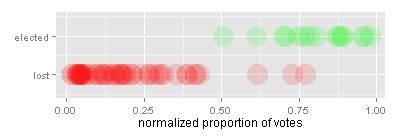圖一：1998-2008 年立法會選舉各候選名單的規範得票率圖二：用概率曲線稱合 1998-2008 年的規範得票率

$f(v') = \frac{1}{1+\exp(7.899974-12.664143v')}.$圖三：從九月七日民調所得的民主黨勝算曲線

1. （全攻型）務求全取兩席。
2. （防守型）確保一席，並伺機提高另一張名單的勝算，情況轉壞則棄車保帥。圖四：總得票率 0.14，全勝機會 0.5 或以上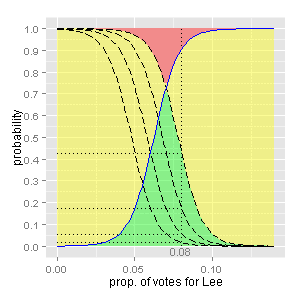圖五：民主黨的全勝機會，對總得票率十分敏感圖六：民主黨的勝算曲線 ($v_\max=0.13$)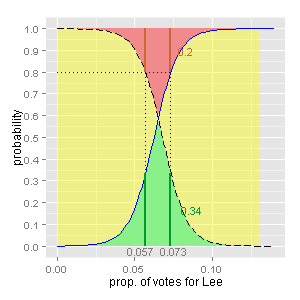圖七：民主黨的勝算曲線 ($v_\max=0.13$)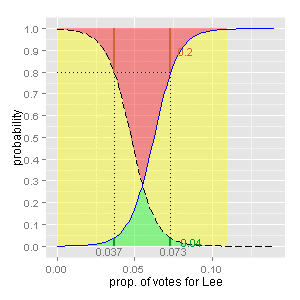圖八：民主黨的勝算曲線 ($v_\max=0.11$)

（至於誰是車，誰是帥，就很難說。我自己就比較希望棄李保陳，但這是另話。）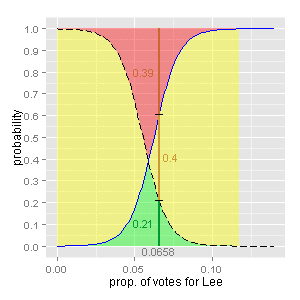圖九：事後孔明圖 ($v_\max=0.1177, v=0.0658$)
Fate/Legco